## Curvature

In general, there are two important types of curvature: Extrinsic Curvature and Intrinsic Curvature. The Extrinsic Curvature of curves in 2- and 3-space was the first type of curvature to be studied historically, culminating in the Frenet Formulas, which describe a Space Curve entirely in terms of its curvature,'' Torsion, and the initial starting point and direction.

After the curvature of 2- and 3-D curves was studied, attention turned to the curvature of surfaces in 3-space. The main curvatures which emerged from this scrutiny are the Mean Curvature, Gaussian Curvature, and the Weingarten Map. Mean Curvature was the most important for applications at the time and was the most studied, but Gaußwas the first to recognize the importance of the Gaussian Curvature.

Because Gaussian Curvature is intrinsic,'' it is detectable to 2-dimensional inhabitants'' of the surface, whereas Mean Curvature and the Weingarten Map are not detectable to someone who can't study the 3-dimensional space surrounding the surface on which he resides. The importance of Gaussian Curvature to an inhabitant is that it controls the surface Area of Spheres around the inhabitant.

Riemannand many others generalized the concept of curvature to Sectional Curvature, Scalar Curvature, the Riemann Tensor, Ricci Curvature, and a host of other Intrinsic and Extrinsic Curvatures. General curvatures no longer need to be numbers, and can take the form of a Map, Group, Groupoid, tensor field, etc.

The simplest form of curvature and that usually first encountered in Calculus is an Extrinsic Curvature. In 2-D, let a Plane Curve be given by Cartesian parametric equations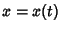and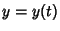. Then the curvature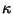is defined by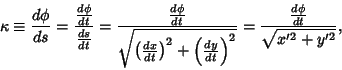(1)

where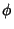is the Polar Angle and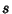is the Arc Length. As can readily be seen from the definition, curvature therefore has units of inverse distance. The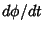derivative in the above equation can be eliminated by using the identity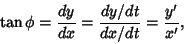(2)

so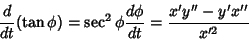(3)

and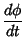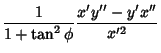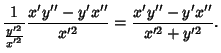(4)

Combining (2) and (4) gives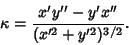(5)

For a 2-D curve written in the form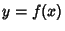, the equation of curvature becomes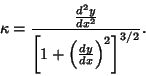(6)

If the 2-D curve is instead parameterized in Polar Coordinates, then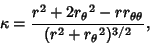(7)

where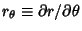(Gray 1993). In Pedal Coordinates, the curvature is given by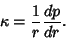(8)

The curvature for a 2-D curve given implicitly by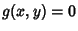is given by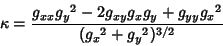(9)

(Gray 1993).

Now consider a parameterized Space Curve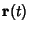in 3-D for which the Tangent Vector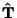is defined as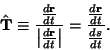(10)

Therefore,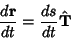(11)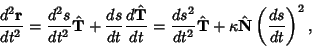(12)

where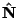is the Normal Vector. But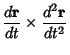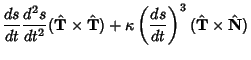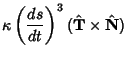(13)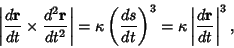(14)

so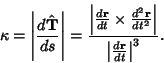(15)

The curvature of a 2-D curve is related to the Radius of Curvature of the curve's Osculating Circle. Consider a Circle specified parametrically by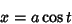(16)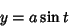(17)

which is tangent to the curve at a given point. The curvature is then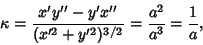(18)

or one over the Radius of Curvature. The curvature of a Circle can also be repeated in vector notation. For the Circle with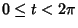, the Arc Length is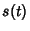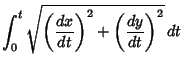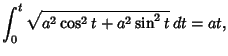(19)

so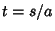and the equations of the Circle can be rewritten as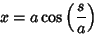(20)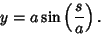(21)

The Position Vector is then given by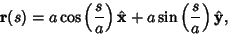(22)

and the Tangent Vector is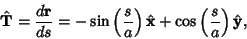(23)

so the curvature is related to the Radius of Curvatureby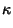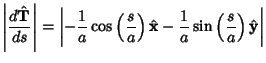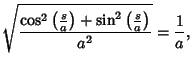(24)

as expected.

Four very important derivative relations in differential geometry related to the Frenet Formulas are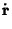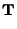(25)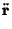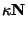(26)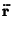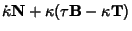(27)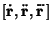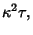(28)

where T is the Tangent Vector, N is the Normal Vector, B is the Binormal Vector, and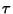is the Torsion (Coxeter 1969, p. 322).

The curvature at a point on a surface takes on a variety of values as the Plane through the normal varies. Asvaries, it achieves a minimum and a maximum (which are in perpendicular directions) known as the Principal Curvatures. As shown in Coxeter (1969, pp. 352-353),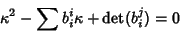(29)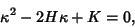(30)

where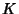is the Gaussian Curvature,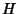is the Mean Curvature, and det denotes the Determinant.

The curvatureis sometimes called the First Curvature and the Torsionthe Second Curvature. In addition, a Third Curvature (sometimes called Total Curvature)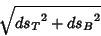(31)

is also defined. A signed version of the curvature of a Circle appearing in the Descartes Circle Theorem for the radius of the fourth of four mutually tangent circles is called the Bend.

See also Bend (Curvature), Curvature Center, Curvature Scalar, Extrinsic Curvature, First Curvature, Four-Vertex Theorem, Gaussian Curvature, Intrinsic Curvature, Lancret Equation, Line of Curvature, Mean Curvature, Normal Curvature, Principal Curvatures, Radius of Curvature, Ricci Curvature, Riemann Tensor, Second Curvature, Sectional Curvature, Soddy Circles, Third Curvature, Torsion (Differential Geometry), Weingarten Map

References

Coxeter, H. S. M. Introduction to Geometry, 2nd ed. New York: Wiley, 1969.

Fischer, G. (Ed.). Plates 79-85 in Mathematische Modelle/Mathematical Models, Bildband/Photograph Volume. Braunschweig, Germany: Vieweg, pp. 74-81, 1986.

Gray, A. Curvature of Curves in the Plane,'' Drawing Plane Curves with Assigned Curvature,'' and Drawing Space Curves with Assigned Curvature.'' §1.5, 6.4, and 7.8 in Modern Differential Geometry of Curves and Surfaces. Boca Raton, FL: CRC Press, pp. 11-13, 68-69, 113-118, and 145-147, 1993.

Kreyszig, E. Principal Normal, Curvature, Osculating Circle.'' §12 in Differential Geometry. New York: Dover, pp. 34-36, 1991.

Yates, R. C. Curvature.'' A Handbook on Curves and Their Properties. Ann Arbor, MI: J. W. Edwards, pp. 60-64, 1952.